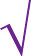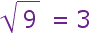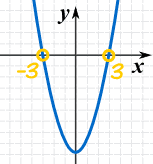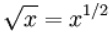# Squares and Square Roots in Algebra

You might like to read our Introduction to Squares and Square Roots first.

## Squares

To square a number, just multiply it by itself ...

### Example: What is 3 squared?

 3 Squared == 3 × 3 = 9

"Squared" is often written as a little 2 like this:This says "4 Squared equals 16"
(the little 2 means the number appears twice in multiplying, so 4×4=16)

## Square Root

A square root goes the other direction:3 squared is 9, so a square root of 9 is 3

What can I multiply by itself to get this?

## Definition

Here is the definition:

A square root of x is a number r whose square is x:

r2 = x
r is a square root of x

## The Square Root SymbolThis is the special symbol that means "square root", it is like a tick, and actually started hundreds of years ago as a dot with a flick upwards. It is called the radical, and always makes mathematics look important!

We can use it like this:we say "square root of 9 equals 3"

### Example: What is √36 ?

Answer: 6 × 6 = 36, so √36 = 6

## Negative Numbers

We can also square negative numbers.

### Example: What is minus 5 squared?

But hang on ... what does "minus 5 squared" mean?

• square the 5, then do the minus?
• or square (−5)?

It isn't clear! And we get different answers:

• square the 5, then do the minus: −(5×5) = −25
• square (−5): (−5)×(−5) = +25

So let's make it clear by using "( )".

### Example Corrected: What is (−5)2 ?

(−5) × (−5) = 25

(because a negative times a negative gives a positive)

That was interesting!

When we square a negative number we get a positive result.

Just the same as when we square a positive number:Now remember our definition of a square root?

A square root of x is a number r whose square is x:

r2 = x
r is a square root of x

And we just found that:

(+5)2 = 25
(−5)2 = 25

So both +5 and −5 are square roots of 25

## Two Square Roots

There can be a positive and negative square root!

This is important to remember.

### Example: Solve w2 = a

w = √a   and   w = −√a

## Principal Square Root

So if there are really two square roots, why do people say √25 = 5 ?

Because means the principal square root ... the one that isn't negative!

There are two square roots, but the symbol means just the principal square root.

### Example:

The square roots of 36 are 6 and −6

But 36 = 6 (not −6)

The Principal Square Root is sometimes called the Positive Square Root (but it can be zero).

## Plus-Minus Sign

 ± is a special symbol that means "plus or minus",
 so instead of writing: w = √a   and   w = −√a we can write: w = ±√a

## In a Nutshell

When we have:r2 = x
then:r = ±√x

## Why Is This Important?

Why is this "plus or minus" important? Because we don't want to miss a solution!

### Example: Solve x2 − 9 = 0

Move 9 to right:x2 = 9
Square Roots:x = ±√9

The "±" tells us to include the "−3" answer also.### Example: Solve for x in (x − 3)2 = 16

Square Roots:x − 3 = ±√16
Calculate √16:x − 3 = ±4
Add 3 to both sides:x = 3 ± 4

Check: (7−3)2 = 42 = 16
Check: (−1−3)2 = (−4)2 = 16

## Square Root of xy

When two numbers are multiplied within a square root, we can split it into a multiplication of two square roots like this:

xy = √xy

but only when x and y are both greater than or equal to 0

### Example: What is √(100×4) ?

√(100×4)= √(100) × √(4)
= 10 × 2
= 20

And xy = √xy :

√8√2= √(8×2)
= √16
= 4

### Example: What is √(−8 × −2) ?

√(−8 × −2) = √(−8) × √(−2)
= ???

We seem to have fallen into some trap here!

We can use Imaginary Numbers, but that leads to a wrong answer of −4

Oh that's right ...

The rule only works when x and y are both greater than or equal to 0

So we can't use that rule here.

Instead just do it this way:

√(−8 × −2) = √16 = +4

### Why does √xy = √x√y ?

We can use the fact that squaring a square root gives us the original value back again:

(√a)2 = a

Assuming a is not negative!

We can do that for xy:(√xy)2 = xy
And also to x, and y, separately:(√xy)2 = (√x)2(√y)2
Use a2b2 = (ab)2:(√xy)2 = (√xy)2
Remove square from both sides:xy = √xy

## An Exponent of a Half

A square root can also be written as a fractional exponent of one-half:but only for x greater than or equal to 0